Next: FILTERS, SIGNALS, AND THEIR Up: Notation Previous: OPERATORS

# SCALARS


n,m,N 		number of components in a vector
x,y,z 		Cartesian coordinatesphase angle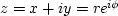complex number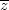complex conjugate of z
t 		time; transmission coefficient
j,k 		index on discrete time
f 		generic function; frequency in cycles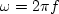angular frequency (common)Z-transform variableconvolution; multiplication (in programs)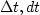sampling time interval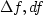frequency sampling intervalextent of time axis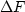extent of frequency axissignal duration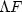spectral bandwidth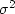variance
c 		reflection coefficientNext: FILTERS, SIGNALS, AND THEIR Up: Notation Previous: OPERATORS
Stanford Exploration Project
10/21/1998